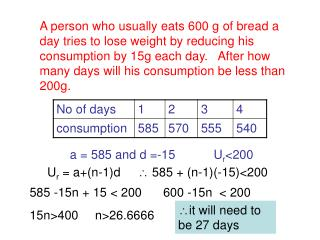DownloadDownload Presentationa = 585 and d =-15

# a = 585 and d =-15

Download Presentation## a = 585 and d =-15

- - - - - - - - - - - - - - - - - - - - - - - - - - - E N D - - - - - - - - - - - - - - - - - - - - - - - - - - -
##### Presentation Transcript

1. A person who usually eats 600 g of bread a day tries to lose weight by reducing his consumption by 15g each day. After how many days will his consumption be less than 200g. a = 585 and d =-15 Ur<200 Ur = a+(n-1)d  585 + (n-1)(-15)<200 585 -15n + 15 < 200 600 -15n < 200 it will need to be 27 days 15n>400 n>26.6666

2. A person who usually eats 600 g of bread a day tries to lose weight by reducing his consumption by 15g each day. After how many days will his total consumption exceed 4500g? a = 585 and d =-15 Sn>4500 Sn=n/2{2a + (n-1)d}  4500 < n/2 {1170+(n-1)(-15)} 4500 < n/2 {1170-15n+15} N >8.8 and <68.2 9000<1170n-15n2+15n 15n2 -1155n + 9000<0 it will need to be 9 days n2 – 77n + 600 < 0